# Campus 101

6864 Registered Allowed team size: 1

This campaign is over.

hackathon
Online
starts on:
Dec 06, 2020, 06:24 PM
ends on:
Dec 06, 2020, 06:25 PM

## Algo - Milestone 2

#### Greedy algorithm

In a game of chess, you think and make decisions about your next move. Whereas, in a game of tennis, your actions are based on the immediate situation. That is, making a decision that seems correct in a particular situation sometimes gives the best solution.

The greedy algorithm is based on such immediate situations.

Greedy strategy

This algorithm works in stages. In each stage, a decision is made that looks like the best solution at a particular point without bothering about the consequences. Thus, it assumes that ‘a local good selection makes for a globally optimal solution’.

The greedy algorithm has two important properties. They are as follows:

• Greedy choice property: This property represents that a globally optimal solution can be obtained by making a locally optimal solution.
• Optimal substructure: If an optimal solution to a problem contains optimal solutions to a subproblem, then this refers to the optimal substructure. This allows you to solve the subproblems and then build up the solution to solve the larger problem.

Applications

• Sorting: Selection sort, topological sort
• Priority queue: Heapsort
• Huffman coding compression algorithm
• Prim’s and Kruskal’s algorithm
• Shortest path in a weighted graph

Let’s understand this concept with a simple example.

You are given a file. You have scanned it and obtained the following data.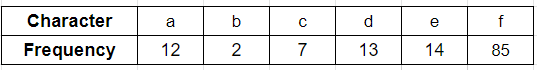The technique

1. You can create a binary tree from each character, Also, this tree can store the frequency with which the characters occur.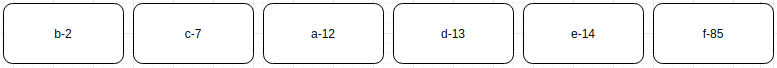1. In the list, find the two binary trees that store the minimum frequency at their nodes.
2. Connect these nodes at a newly created common node that stores the sum of the frequencies of all the nodes connected below it, as shown in the following diagram: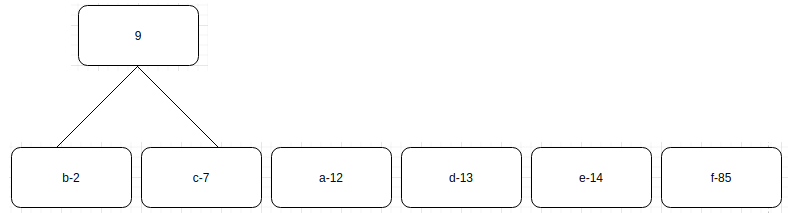4. Repeat this process until one tree is left.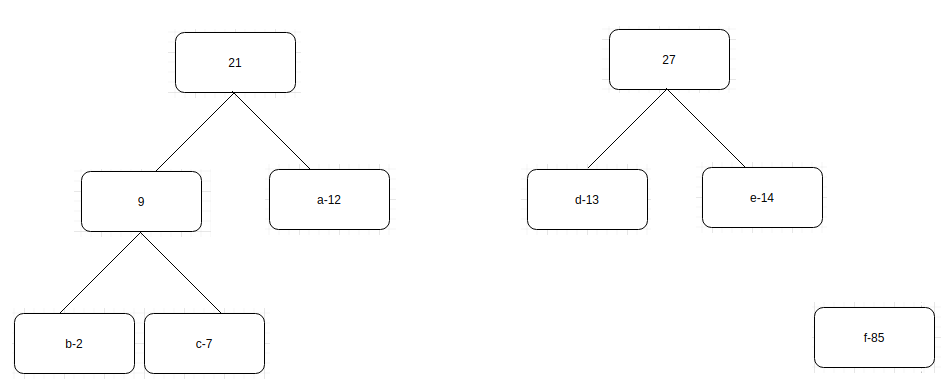5. Finally, you get the following tree.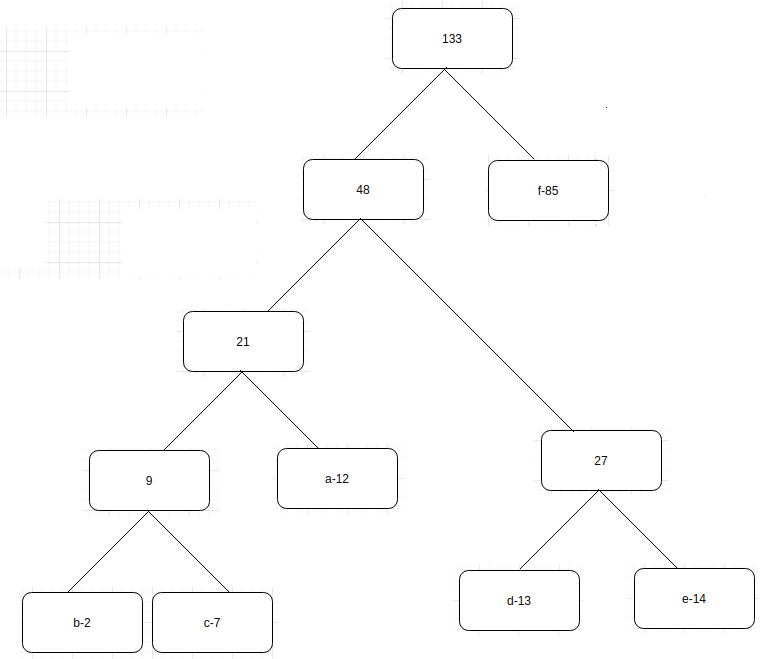1. Once the tree is built, each leaf node corresponds to a letter with a code. To determine this code for a particular node, traverse from the root to the leaf node. For each move to the left, append a 0 to the code and for each move to the right, append a 1. You will obtain the following representation: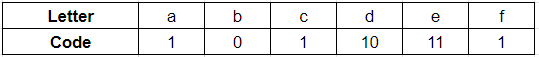This is called the Huffman coding algorithm. The Python implementation of this is as follows: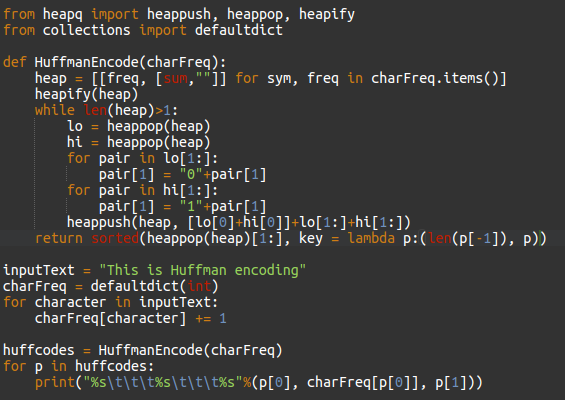Calculating the number of bits saved

Let’s assume each character is stored with a three-bit code. Since there are 133 such characters, the total number of bits used is 3 * 133 = 399 bits. Thus, originally, the same task provided will consume 399 bits.

Using Huffman coding, the number of bits used is 238 (calculation is shown in the following table:)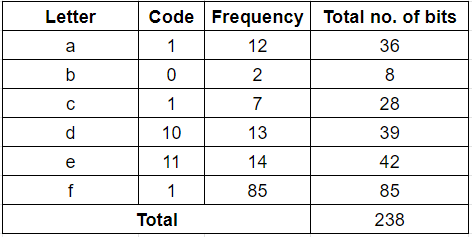Hence, you save 399-238 = 161 bits or approximately 40% of the storage place.

You can refer to example 1 and example 2 to study about Huffman coding.

Try the following question:

You are given an array F with size n. Assume the array content F[i] represents the length of the i^{th} file. Your task is to merge all these files into a single file.

Also, check if the following algorithm is the best solution to this problem:

Merge the files contiguously. In other words, select the first two files and merge them. Then, select the output you obtained and combine it with the third file, and so on.